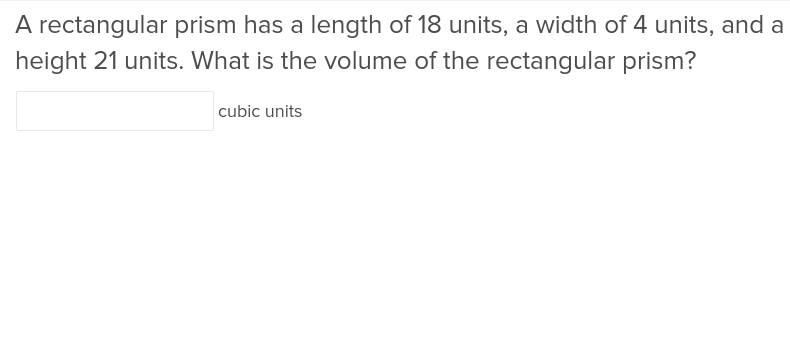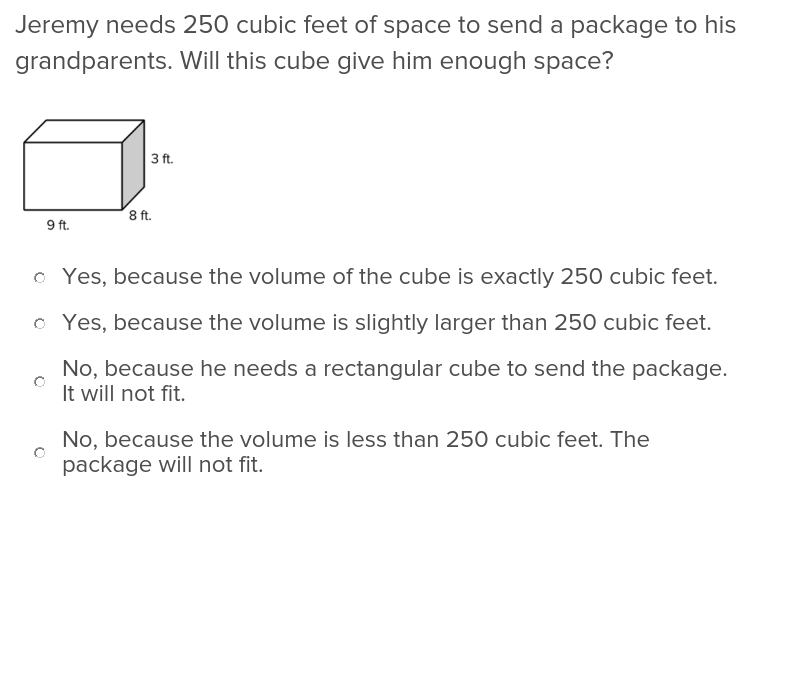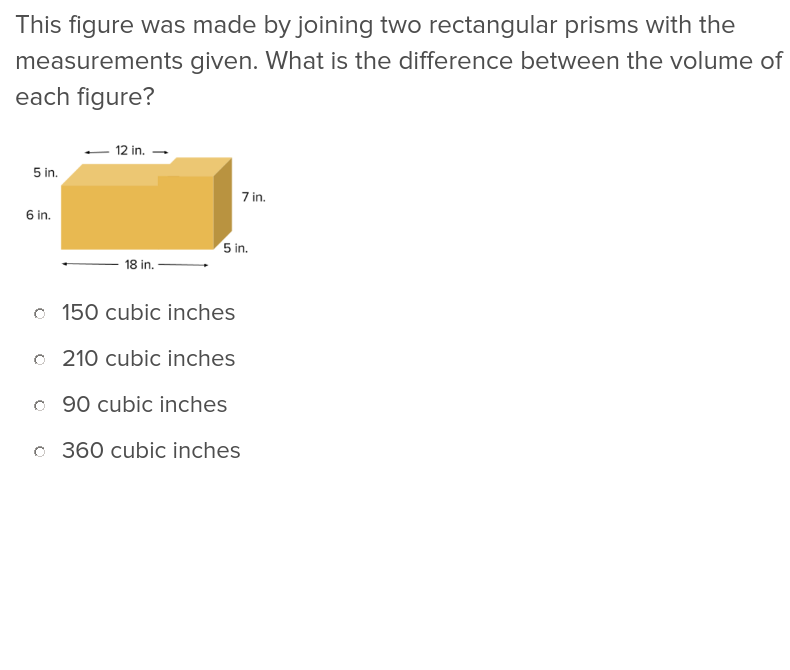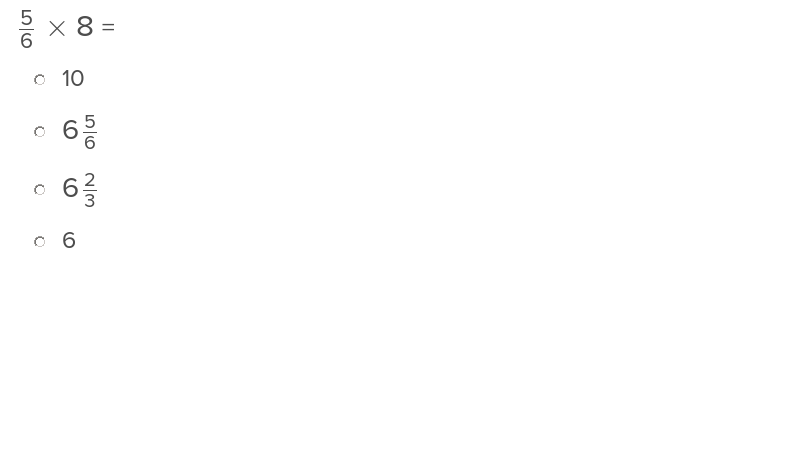Exercises

# Measurement 2

Calculating the volume of rectangular prisms is a new skill that is introduced in 5th grade. Students will apply mathematical formulas to find the volume of different kinds of figures and also determine the volume of a figure composed of two connected rectangular prisms. Learners will also work with other kinds of measurement when they make larger or smaller versions of figures (to scale) and learn to convert measurements.
This lesson includes 5 printable learning activities.

## Volume of a Rectangular PrismWith a clear illustration and instructions, this exercise will teach students how to calculate the seemingly complex volume of a rectangular prism.

## Formulas for Calculating VolumeTeach students how to calculate volume by exposing them to formulas they can use on their own.Students will be able to put the new skills they gain from this exercise to use whenever they want to add volumes together.

## Scaling Shapes to a Whole Number FactorGive students the ability to scale shapes to a whole number factor with a little hands-on learning through this exercise.